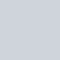# Difference between Plane and Geodetic Surveying

 Plane Surveying Geodetic Surveying Curvature of the earth is not taken in to consideration In this curvature of earth is considered. In plane Surveying, line joining any two points of triangle formed by any three points is considered as straight line and plane triangle are assumed to be plane angles. In geodetic surveying line joining two points of triangle formed by three points is considered as curved line of spherical triangle and angles of triangle are considered as spherical angles. This survey is done on smaller area less than 250km2 This survey is done on large area greater than 250 km2 Required accuracy is competitively low. High accuracy is required Simple methods and instruments can be used as the required accuracy is low. Very refined methods and instruments are used.

Source